Back to Kemp Acoustics HomeNext: Multimodal input impulse response Up: Multimodal reflections Previous: Multimodal reflectance matrix   Contents

# Multimodal reflectance of a single discontinuity

The simplest case of a multimodal calculation of the reflectance matrix is for the reflection of plane waves from a single discontinuity in cross-section between two semi-infinite cylinders. We will define the plane on the left of the discontinuity as plane 1 and the plane on the right as plane 2 (see figure 2.2). If a plane sine-wave is incident from the left, it will be partially reflected and partially transmitted at the boundary. The impedance matrix on the right hand side of the boundary will be equal to the characteristic impedance matrix because only forward propagating waves are present there:
(6.9)

where
is simply the characteristic impedance matrix defined in equation (2.42) when
. This matrix can then be projected to get the impedance matrix on the left by substitution into equation (2.97):(6.10)

The reflectance matrix at surface 1 can then be found from equation (6.8) by substituting in
from equation (6.10), also noting that
is the characteristic impedance matrix on surface 1 with
:(6.11)

If particular values of the cylinder radii,
andare chosen, the reflectance matrix can be calculated for a number of frequency values. However, as with the radiation impedance calculations in chapter 3, the results may be presented in a form valid for all radius values by reformulating the equations in terms of the dimensionless frequency variable
and the radius ratio
. The characteristic impedance of theth mode on the left of the discontinuity becomes
where. Dividing by
normalises the characteristic impedance of theth mode to give
(6.12)

Similarly, the normalised characteristic impedance of theth mode on surface 2 is
(6.13)

using
. The reflectance matrix is then
(6.14)

where
andare diagonal matrices with the entries on theth diagonals given by equations (6.12) and (6.13) respectively. The reflectance is now a function of the dimensionless variablesand
only.

Before proceeding to produce graphs from the reflectance matrix we should consider which elements determine the reflected sound. For our example we have a plane sine-wave of unit amplitude incident on the discontinuity from the left. This forward travelling wave is represented by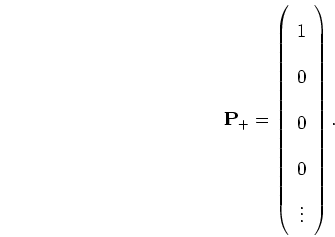(6.15)

From equation (6.7) the reflection is then(6.16)

The plane wave component of the reflection is therefore simply given by the corner value of the reflectance matrix,. We will refer to thevalue as the plane reflectance. Although we will discuss only the plane reflection from an incident plane wave, it is important to note that we are doing a multimodal calculation. Depending on the frequency, some of the incident energy is converted into the other modes. In turn the plane reflectance will vary with frequency.

Figure 6.1 displays the absolute value of the plane reflectance for radius ratio of
against the dimensionless frequency variable,as a green line. At low frequencies the plane reflectance matches the plane wave reflection coefficient of equation (2.20) which is
for. The maximum in the graph corresponds to the cut-off of the first non-plane mode in the larger of the ducts. A blue line shows the absolute value of the plane reflectance from the inductance method published by Kergomard and Garcia . The use of the inductance method to calculate reflectance is summarised in appendix C. The inductance method is designed to be accurate in the region, ie.in this example. Agreement between the inductance method and the current multimodal method is very good in this region.

Figure 6.2 shows the plane reflectance decaying to zero at high frequencies. We can understand this by noting that high frequencies do not experience much diffraction meaning almost 100of the energy is transmitted across an expansion.

Figure 6.3 shows the plane reflectance against frequency for a number of different radius ratios. The low frequency limit shows that large cross-section changes reflect more energy in agreement with the plane wave approximation reflection coefficient. Large cross-section changes also lead to a quicker drop off of plane reflectance with frequency. The detail in the graphs can be understood by considering the cut-off frequencies in the smaller pipe (section 1) and the larger pipe (section 2). The cut-off wavenumber for themode in section 1 is
and the
cut-off in section 1 is
. We can see all the graphs have small minima at these values. The large peaks, however, are due to the cut-off frequencies in the pipe on the right.

In order to show the cut-off frequencies on surface 2, the graphs are replotted using a dimensionless frequency variable of
. The resulting plot is shown in figure 6.4. The lowest cut-off wavenumber present is for themode in section 2 with a dimensionless frequency of. All the spectra show peaks at this point because above this frequency energy is absorbed from the plane reflection by transmission into the propagating mode. The reduction in reflected amplitude after thecut-off frequency is particularly marked in thecase. The pressure profile of themode on
has a circular amplitude maximum in the centre taking up an area similar to the pressure amplitude of the plane mode on
. Since the pressure profile onmust match the pressure profile on, above the cut-off frequency strong transmission of the propagatingmode is favoured. Themode in section 2 is responsible for the peaks at
in the plane reflectance spectra.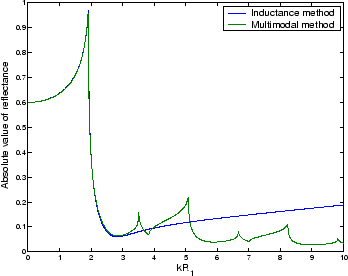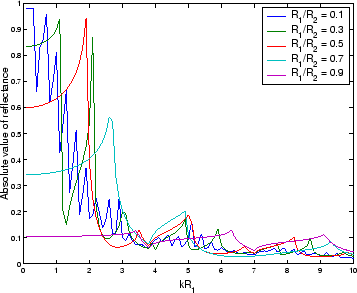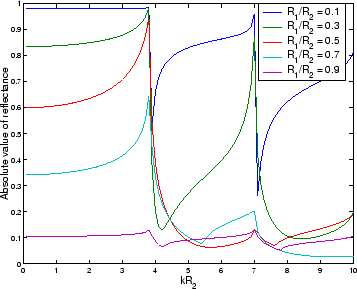Back to Kemp Acoustics HomeNext: Multimodal input impulse response Up: Multimodal reflections Previous: Multimodal reflectance matrix   Contents
Jonathan Kemp 2003-03-24